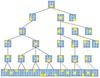dfs 订阅

Depth-First-Search

• ## DFS

千次阅读 2017-12-07 12:56:41
一、DFS介绍  深度优先搜索算法（英语：Depth-First-Search，简称DFS）是一种用于遍历或搜索树或图的算法。沿着树的深度遍历树的节点，尽可能深的搜索树的分支。当节点v的所在边都己被探寻过，搜索将回溯到发现...
一、DFS介绍

深度优先搜索算法（英语：Depth-First-Search，简称DFS）是一种用于遍历或搜索树或图的算法。沿着树的深度遍历树的节点，尽可能深的搜索树的分支。当节点v的所在边都己被探寻过，搜索将回溯到发现节点v的那条边的起始节点。这一过程一直进行到已发现从源节点可达的所有节点为止。如果还存在未被发现的节点，则选择其中一个作为源节点并重复以上过程，整个进程反复进行直到所有节点都被访问为止。属于盲目搜索。

深度优先搜索是图论中的经典算法，利用深度优先搜索算法可以产生目标图的相应拓扑排序表，利用拓扑排序表可以方便的解决很多相关的图论问题，如最大路径问题等等。

1.1 主要思想

主要思想：不撞南墙不回头。

深度优先遍历的主要思想就是：首先以一个未被访问过的顶点作为起始顶点，沿当前顶点的边走到未访问过的顶点；当没有未访问过的顶点时，则回到上一个顶点，继续试探访问别的顶点，直到所有的顶点都被访问。沿着某条路径遍历直到末端，然后回溯，再沿着另一条进行同样的遍历，直到所有的顶点都被访问过为止。

1.2 伪代码

 void dfs()
{
// 判断是否到达终点
if() {
return;
}

// 尝试每一个可走方向（右下左上）
for(i=0; i<n; i++){
// 判断是否可走,可走调用递归尝试下一步，不可走则尝试该点的其他方向
if () {
// 继续下一步
dfs();
}
}
}
1.3 C++代码

   public void DFS( int x, int y )
throws Exception
{

int tx, ty;

int[] pos =
{ x, y };

// 是否到达目的地
if (mMapView[y][x] == 8)
{
throw new Exception("find");
}

// 顺时针循环，右下左上四个方向
for (int k = 0; k < 4; k++)
{
tx = x + next[k];
ty = y + next[k];

// 是否出了边界
boolean isOut = tx < 0 || tx >= mapWidth || ty < 0 || ty >= mapHeight;
if (!isOut)
{

// 是否是障碍物
if (mMapView[ty][tx] == 0 && dfs_book[tx][ty] == 0 || mMapView[ty][tx] == 8)
{
dfs_book[tx][ty] = 1;
DFS(tx, ty);
dfs_book[tx][ty] = 0;
}
}

}
}

// 判断方向的数组 int[][] next =
{
{ 0, 1 }, // 右
{ 1, 0 }, // 下
{ 0, -1 }, // 左
{ -1, 0 } // 上
};

二、实现方法

1. 首先将根节点放入队列中。

2. 从队列中取出第一个节点，并检验它是否为目标。

如果找到目标，则结束搜寻并回传结果。

否则将它某一个尚未检验过的直接子节点加入队列中。

3. 重复步骤2。

4. 如果不存在未检测过的直接子节点。

将上一级节点加入队列中。

重复步骤2。

5. 重复步骤4。

6. 若队列为空，表示整张图都检查过了——亦即图中没有欲搜寻的目标。结束搜寻并回传“找不到目标”。

C++的实现

定义一个结构体来表达一个二叉树的节点的结构：

1 struct Node {
2     int self;     // 数据
3     Node *left;   // 左节点
4     Node *right;  // 右节点
5 };

那么我们在搜索一个树的时候，从一个节点开始，能首先获取的是它的两个子节点。例如：

A
B           C
D   E          F   G

A是第一个访问的，然后顺序是B和D、然后是E。然后再是C、F、G。那么我们怎么来保证这个顺序呢？

这里就应该用堆栈的结构，因为堆栈是一个先进后出的顺序。通过使用C++的STL，下面的程序能帮助理解：

const int TREE_SIZE = 9;
3 std::stack<Node *> unvisited;
4 Node nodes[TREE_SIZE];
5 Node *current;
6
7 //初始化树
8 for (int i = 0; i < TREE_SIZE; i++) {
9     nodes[i].self = i;
10     int child = i * 2 + 1;
11     if (child < TREE_SIZE) // Left child
12         nodes[i].left = &nodes[child];
13     else
14         nodes[i].left = NULL;
15     child++;
16     if (child < TREE_SIZE) // Right child
17         nodes[i].right = &nodes[child];
18     else
19         nodes[i].right = NULL;
20 }
21
22 unvisited.push(&nodes); //先把0放入UNVISITED stack
23
24 // 只有UNVISITED不空
25 while (!unvisited.empty()) {
26     current = (unvisited.top()); //当前应该访问的
27     unvisited.pop();
28     if (current->right != NULL)
29         unvisited.push(current->right); // 把右边压入 因为右边的访问次序是在左边之后
30     if (current->left != NULL)
31         unvisited.push(current->left);
32     cout << current->self << endl;
33 }
参考： http://blog.csdn.net/g11d111/article/details/75645970


展开全文• Consider a Depth-First-Search(DFS) spanning tree T of a undirected connected graph G, we define a T-Simple Circle as a path v1, v2, ..., vk (v1 = vk) in G that contains at most one edge which not ...as each lines path
• ## DFS.zip 写号工具

热门讨论 2013-01-27 00:29:43
DFS CDMA Tool多功能的DFS文件固件下载到设备或做一些诸如reflesh的ESN/ MEID修复操作或解锁硬盘。 DMF文件可以帮助你做： 硬盘解锁 固件更新或修复 更改语言包 ESN/ MEID/ IMEI修复 拨号面板*#7284#，USB改成...
• DFS IDFS 离散傅里叶级数
                             DFS IDFS  离散傅里叶级数
要将信号进行时域到频域的变换，那么就离不开dfs  要变回去又离不来 Idfs
下面就是 matlab 实现的两个函数

很简单 用公式
下面是代码：
function [Xk] = dfs(xn,N )
n = [0: 1: N-1];
k = n;
WN = exp(-j*2*pi/N);
nk = n'*k ;
WNnk = WN.^nk;
Xk = xn*WNnk;
end
下面是idfs代码：

function[xn] = idfs(Xk, N)
n = [0 :1: N-1] ;
k = n;
WN = exp(-j*2*pi/N);
nk = n'*k;
WNnk = WN.^(-nk);
xn = Xk* WNnk/N;
end其应用 给出周期方波
x(n) =    1                 mN <=  n <= mN + L -1
0                mN  + L <= n <= (m+1)N -1            m 为0  -1 +1 。。。。。
Ｌ／Ｎ为占空比   给出X (k) 的曲线
这里要注意的是要进行fftshift  搬移之后才会形成对称的图形
才能形成 k = [-N / 2 : N /2] ;  且没有那么长那就用0 补
代码中一共实现了四种长不同L值的变换
代码实现：
clf ;
L = 5 ;
N = 20 ;
xn  =[ones(1,L) zeros(1,N-L)];
k = [-N/2:N/2];
Xk = dfs(xn, N);
magXk = abs([Xk(N/2 + 1:N ), Xk(1:N/2+1)]);
subplot(221);
stem(k, magXk);
xlabel('k');
ylabel('Xk');
title('L = 5 , N = 20 wave ');

L = 5 ;
N = 40 ;
xn  =[ones(1,L) zeros(1,N-L)];
k = [-N/2:N/2];
Xk = dfs(xn, N);
magXk = abs([Xk(N/2 + 1:N ), Xk(1:N/2+1)]); % 这个的目的就有点类似于fftshift
subplot(222);
stem(k, magXk);
xlabel('k');
ylabel('Xk');
title('L = 5 , N = 40 wave ');

L = 6 ;
N = 40 ;
xn  =[ones(1,L) zeros(1,N-L)];
k = [-N/2:N/2];
Xk = dfs(xn, N);
magXk = abs([Xk(N/2 + 1:N ), Xk(1:N/2+1)]);
subplot(223);
stem(k, magXk);
xlabel('k');
ylabel('Xk');
title('L = 6 , N = 40 wave ');

L = 7;
N = 20 ;
xn  =[ones(1,L) zeros(1,N-L)];
k = [-N/2:N/2];
Xk = dfs(xn, N);
magXk = abs([Xk(N/2 + 1:N ), Xk(1:N/2+1)]);
subplot(224);
stem(k, magXk);
xlabel('k');
ylabel('Xk');
title('L = 7 , N = 20 wave ');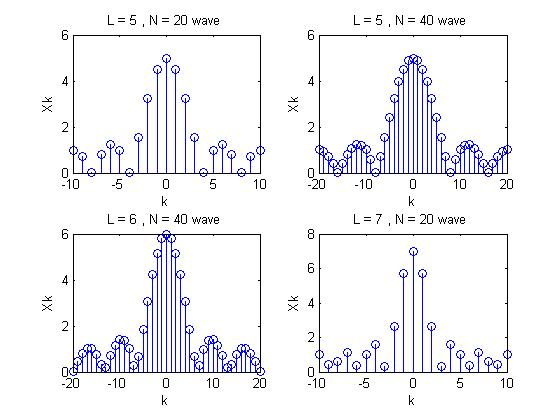展开全文• ## DFS原理白话解析

万次阅读 多人点赞 2018-11-04 11:20:57
看了许多的关于dfs的博客，自己也研究了好多遍，最终算是入门了，下面就简单的个人理解的原理以及结合一个简单的全排列实例进行讲解。 原理简介 dfs基于递归思想，递归思想就是把一个事拆分成若干见相同的小事共同...
前言
看了许多的关于dfs的博客，自己也研究了好多遍，最终算是入门了，下面就简单的个人理解的原理以及结合一个简单的全排列实例进行讲解。
原理简介
DFS基于递归思想，递归思想就是把一个事拆分成若干见相同的小事共同组合完成，具体见下图的斐波那契的图文解决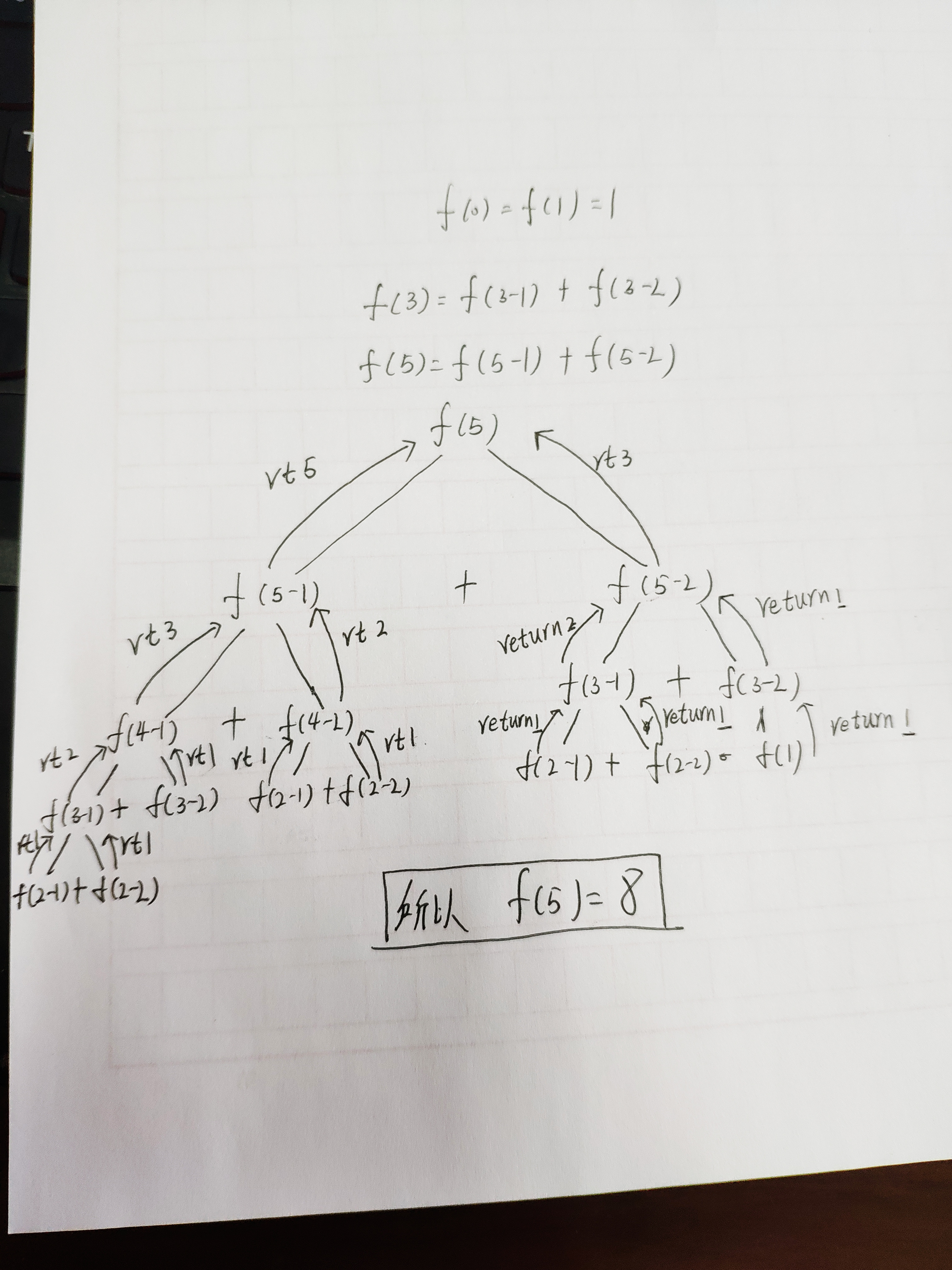这就是一个最典型的递归，入口f(5)出口就是每次递归的return；
说完递归就是dfs，其有两个重要的标志，也就是两个数组，一个用来标记该点是否被访问过，一个用来把该点放入数组，所以这两个标记是相辅相成的，一定同时出现；dfs就是随机选定一个起点将其标记为已经访问过的点，然后就是递归调用进行与其相邻的点的搜索，直到所有的点都被访问完。
话不多说上例子
全排列，也就是1-n，输出其所有的排列结果。
代码如下，接下来会详细解读代码：
#include <stdio.h>
#include <iostream>
using namespace std;
int a,b,n;
void print()
{
int i;
for(i=1;i<=n;i++)
{
cout<<a[i]<<' ';
}
cout<<endl;
}
inline void dfs(int i)
{
int j;
if(i==n+1)
{
print();
return;
}
for(j=1;j<=n;j++)
{
if(b[j]==0)
{
a[i]=j;
b[j]=1;
dfs(i+1);
b[j]=0;
}
}
}
int main()
{
ios::sync_with_stdio(false);
cin>>n;
dfs(1);
return 0;
}


注：以上代码来自csdn博客  Apro1066。
这个代码看上去不多，但是确实经典，有好多值得推敲的东西。
首先主函数中的dfs(1)这是dfs函数进入，传入参数1就是从1开始，主要在dfs函数的理解，下面的图片展示了展示了这个过程。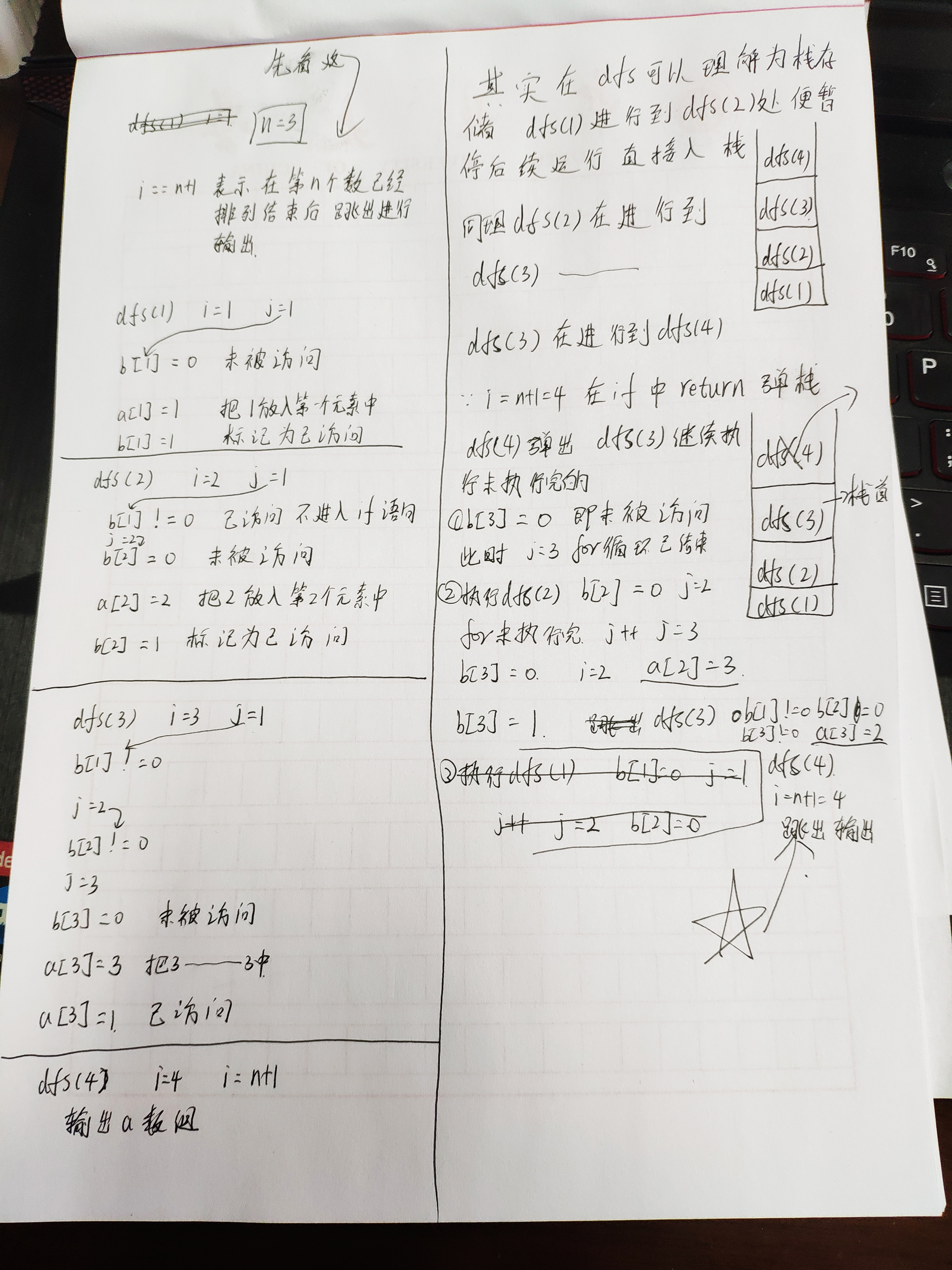下面是一个DFS的经典问题-走迷宫,大家可以结合代码做进一步的理解。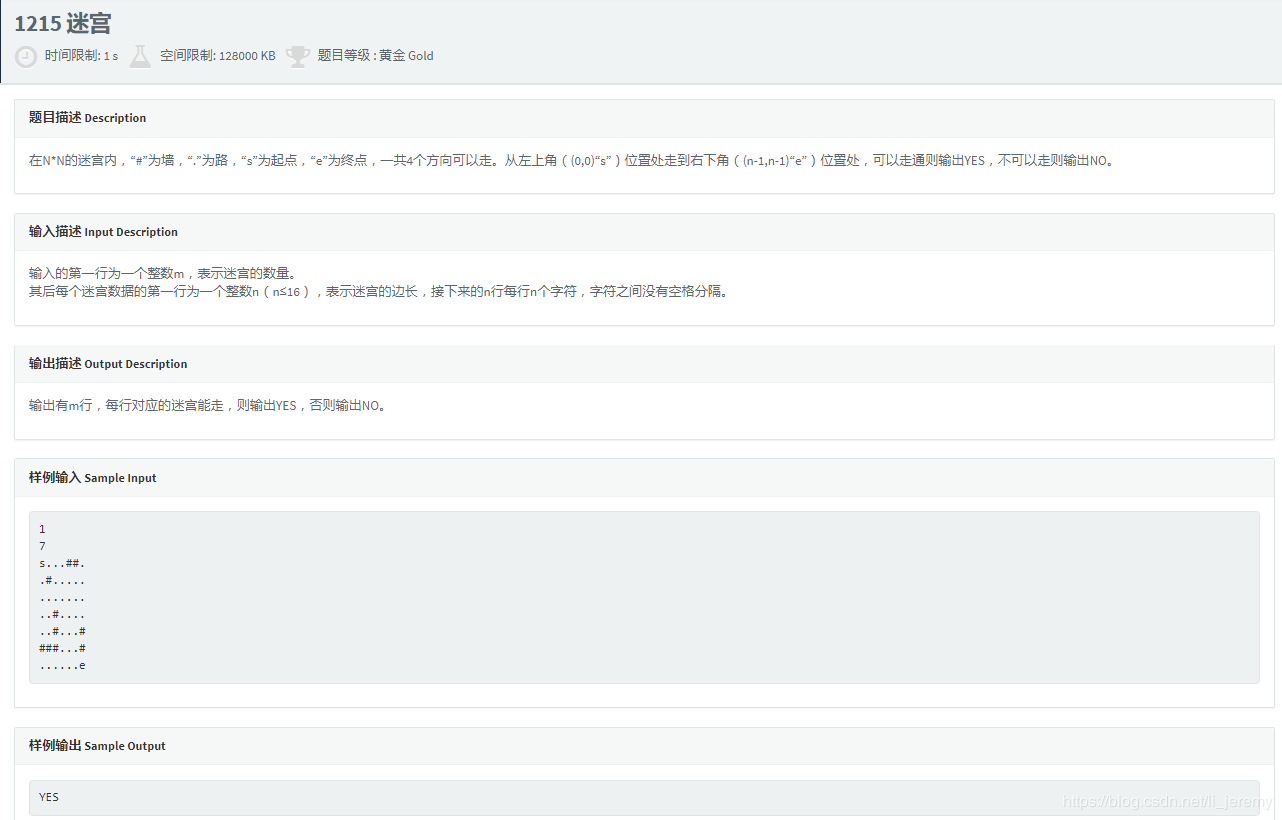Code
#include<bits/stdc++.h>
using namespace std;
bool a={0};
char b;   //存放迷宫
int flag=0;     //如果能走出去就标记为1，反之为0
int xx={0-1,0,1},yy={-1,0,1,0};
int n,kx,ky;   //n是迷宫的边长，kx是x进行加减之后的值，同理ky。
void dfs(int x,int y){
for(int i=0;i<=3;i++){
kx=x+xx[i];
ky=y+yy[i];
if((b[kx][ky] == '.'||b[kx][ky] == 'e')&&kx>=0&&ky>=0&&kx<n&&ky<n&&a[kx][ky]==0){
a[kx][ky]=1;
if(b[kx][ky] == 'e'){
flag = 1;
}else{
dfs(kx,ky);
}
}
}
}

int main(){
int k=1,sum;
cin>>sum;
while(k<=sum){
memset(a,0,sizeof(a)); //将标记数组a全部记为 0，表示这个点未走过
cin>>n;
for(int i = 0;i<n;i++){
for(int j=0;j<n;j++)
cin>>b[i][j];
}
for(int i = 0;i<n;i++){
for(int j=0;j<n;j++){
if(b[i][j]=='s')
dfs(i,j);
}

}
if(flag==1){
cout<<"YES"<<endl;
}else{
cout<<"NO"<<endl;
}
k++;
}
return 0;
}

有不懂的地方或者有错误的地方欢迎评论批评指正。


展开全文全排列
• 搭建了一个hadoop集群，用hdfs dfs -ls /命令，列出的是本地系统的根目录。 用hdfs dfs -ls hdfs://servicename/ 列出的目录才是hdfs上的目录，可能是什么原因？ 执行hive创建的目录也是在本地系统目录上。 集群的...hadoop集群 hdfs 格式化
• 掌握 Windows Server 2016 分布式网络服务的应用场景，理解 DFS 命名空间，复制，BranchCache 的基本组件，工作原理，能够规划，部署和配置多站点和多分支机构场景中，DFS 命名空间服务，DFS 复制和 BranchCache ...
• ## BFS 、DFS区别，详解

万次阅读 多人点赞 2017-08-18 21:14:36
BFS 、DFS区别，详解写在最前的三点： 1、所谓图的遍历就是按照某种次序访问图的每一顶点一次仅且一次。 2、实现bfs和dfs都需要解决的一个问题就是如何存储图。一般有两种方法：邻接矩阵和邻接表。这里为简单起 ...
BFS 、DFS区别，详解
写在最前的三点：
1、所谓图的遍历就是按照某种次序访问图的每一顶点一次且仅一次。
2、实现bfs和dfs都需要解决的一个问题就是如何存储图。一般有两种方法：邻接矩阵和邻接表。这里为简单起见，均采用邻接矩阵存储，说白了也就是二维数组。
3、本文章的小测试部分的测试实例是下图，图G。
4、代码里store_graph部分没有实现该图，按编号自己输进去吧。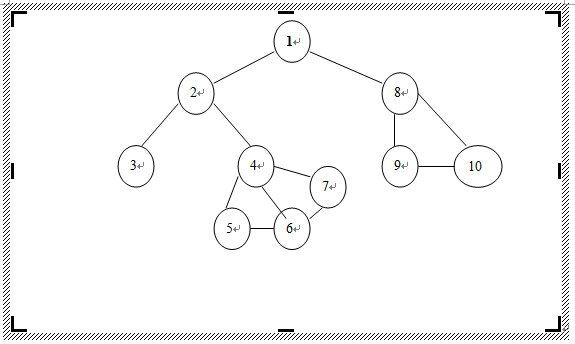一、深度优先搜索遍历
1、从顶点v出发深度遍历图G的算法
① 访问v
② 依次从顶点v未被访问的邻接点出发深度遍历。
2、一点心得：dfs算法最大特色就在于其递归特性，使得算法代码简洁。但也由于递归使得算法难以理解，原因
在于递归使得初学者难以把握程序运行到何处了！一点建议就是先学好递归，把握函数调用是的种种。
3、算法代码：
#include<iostream>
#include<string.h>
using namespace std;
int a;
bool visited;

void store_graph()  //邻接矩阵存储图
{
int i,j;

for(i=1;i<=10;i++)
for(j=1;j<=10;j++)
cin>>a[i][j];
}

void dfs_graph()    //深度遍历图
{
void dfs(int v);

memset(visited,false,sizeof(visited));

for(int i=1;i<=10;i++)  //遍历每个顶点是为了防止图不连通时无法访问每个顶点
if(visited[i]==false)
dfs(i);
}

void dfs(int v)  //深度遍历顶点
{

cout<<v<<" ";  //访问顶点v
visited[v]=true;

{

}
}

{
for(int i=1;i<=10;i++)
if(a[x][i]==1 && visited[i]==false)
return i;

return 0;
}

int main()
{
cout<<"初始化图:"<<endl;
store_graph();

cout<<"dfs遍历结果:"<<endl;
dfs_graph();

return 0;
}


4、小测试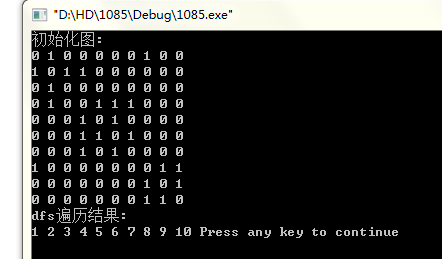二、广度优先搜索遍历
1、从顶点v出发遍历图G的算法买描述如下：
①访问v
②假设最近一层的访问顶点依次为vi1,vi2,vi3…vik，则依次访问vi1,vi2,vi3…vik的未被访问的邻接点
③重复②知道没有未被访问的邻接点为止
2、一点心得：bfs算法其实就是一种层次遍历算法。从算法描述可以看到该算法要用到队列这一数据结构。我这
里用STL中的实现。该算法由于不是递归算法，所以程序流程是清晰的。
3、算法代码：

#include<iostream>
#include<queue>
#include<string.h>
using namespace std;

int a;
bool visited;

void store_graph()
{
for(int i=1;i<=10;i++)
for(int j=1;j<=10;j++)
cin>>a[i][j];
}

void bfs_graph()
{
void bfs(int v);

memset(visited,false,sizeof(visited));

for(int i=1;i<=10;i++)
if(visited[i]==false)
bfs(i);
}

void bfs(int v)
{

queue<int> myqueue;

cout<<v<<" ";
visited[v]=true;
myqueue.push(v);

while(!myqueue.empty())    //队列非空表示还有顶点未遍历到
{
temp=myqueue.front();  //获得队列头元素
myqueue.pop();         //头元素出队

{
{
}

}
}
}

{
for(int i=1;i<=10;i++)
if(a[x][i]==1 && visited[i]==false)
return i;

return 0;
}

int main()
{
cout<<"初始化图:"<<endl;
store_graph();

cout<<"bfs遍历结果:"<<endl;
bfs_graph();

return 0;
}


4、小测试：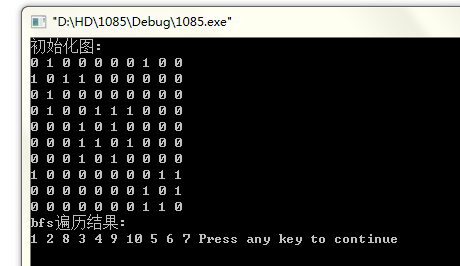展开全文遍历 bfs
• ## POJ 1062 DFS

千次阅读 2012-07-30 22:18:49
感觉自己已经有N久没有写过DFS算法了，因为这道题目在DFS的过程中会保留和计算一些值，所以导致程序看起来有点丑陋。话不多说 贴题说思路，目前水平有限，发博客的主要原因是要写给自己，作为一段时间学习的印证吧。...struct 算法
• DFS的递归算法中，DFS框架如下： 1访问起点v0 2依次以v0的未访问的连接点为起点，DFS搜索图，直至图中所有与v0路径相通的顶点都被访问。 3若该图为非连通图，则图中一定还存在未被访问的顶点，选取该顶点为起点...数据结构
• 'Hadoop DFS'和'Hadoop FS'的区别 While exploring HDFS, I came across these two syntaxes for querying HDFS: > hadoop dfs > hadoop fs whyhdfs hadoop
• ## DFS理解(java)

万次阅读 2020-03-30 16:09:51
DFS（深度优先搜索） /* DFS（深度优先搜索），“深度”是关键，以走迷宫为例，不撞南墙不回头，先找到最深的，然后回溯到上一个路口，以此类推。 有上述可知，DFS可由递归实现，所谓的南墙就是递归边界。 */ //...算法
• // /*#include #include #define MAX 100 bool mark[MAX];//标记数组 //定义邻接矩阵 typedef struct { int n,e; int vexs[MAX]; int edge[MAX][MAX];...void dfsm(int i) { int j;graph matrix struct 算法
• ## BFS和DFS算法原理（通俗易懂版）

万次阅读 多人点赞 2016-11-16 17:25:32
DFS 算法 思想：一直往深处走，直到找到解或者走不下去为止 BFS算法 DFS：使用栈保存未被检测的结点，结点按照深度优先的次序被访问并依次被压入栈中，并以相反的次序出栈进行新的检测。 BFS：使用队列...bfs 算法

千次阅读 2018-03-29 21:14:45Hadoopc++
• void dfs(int i,int cnt) { if(i == 0) { ans = max(ans,cnt); return ; } if(ans == V || cnt+sum[i] ) //cut return ; if(cnt+w[i] ) dfs(i-1,cnt+w[i]); dfs(i-1,cnt); } int main() { int t; scanf("%d",&t); ...c++
• void dfs(int a,int b); int main() { int i,j,z = 0; scanf("%d %d",&n,&m); for(i = 0;i ; i++) { for(j = 0;j ; j++) { scanf(" %c",&water[i][j]); } } for(i = 0;i ; i++) { for(j = 0;j ; ...c++
• ## C++dfs

千次阅读 2018-08-01 12:06:44
如果学过数据结构，或者看过一些其他的资料，你会发现很多书上都会把栈和其他数据结构一起讲解，为什么我们会把栈放到深度优先搜索呢。到目前为止，我们还没有提及到深度优先搜索。没关系，你先好好理解栈和递归，...
• ## WIFI DFS测试介绍

千次阅读 2016-02-29 10:31:47
1. 概述:  目前在802.11系列标准中，涉及物理层的有4个标准：802.11、802.11b、802.11a、802.11g。根据不同的物理层标准，无线局域网设备通常被归为不同的类别，如常说的802.11b无线局域网设备、802.11
• ## BFS与DFS总结

千次阅读 2014-07-20 23:05:10
• ## 八皇后问题 DFS

千次阅读 2014-05-06 22:36:22
• /* ... 题目大意：找出仅用0和1 组成的十进制的数构成是出入n的倍数（n  解题思路：有人直接打表打了200个，因为并不像想的那样数据那么大，有的直接用数学原理。... 当然我用了dfs深搜从第一位1开始往下搜，而且是暴搜索
• ## DFS入门

千次阅读 2018-09-09 10:41:00
因为感觉自己学艺不精啊，BFS和DFS都是一种搜索算法，我感觉BFS没有那么难，因为他比较好理解，但是DFS因为牵扯到递归和回溯等，不太好理解，但是他的实用性是毋庸置疑的，所以即使这个算法再难，我们还是要试着去......